# How do you round 265,427 to the nearest ten thousand?Kaley

Solution:

Step:1 The given number is 265,427.

expand the number in power of 10 as shown below: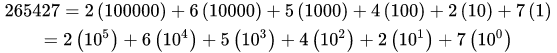To round of the number nearest to 10000 or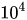, analyze the coefficient of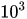,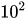,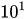and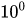.

Step:2 If coefficient ofis greater than 5 then increase the coefficient of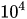and eliminate the coefficients,andotherwise eliminate the coefficients,and, to round of the number nearest to ten thousand.

Step:3 But here the coefficient ofis equal to 5, in this case check the coefficient of, if it is other than 0 then increase the coefficient ofand eliminate the coefficients,and, to round of the number nearest to ten thousand.

Step:4 Here, coefficient ofis 4, therefore increase the coefficient ofand eliminate the coefficients,and, to round of the number nearest to ten thousand as follows:Thus, the number 265,427 round to the nearest ten thousand is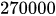.

The number 265,427 round to the nearest ten thousand is.

Similar Problems:

Problem 1:

Write the number 234 to nearest tenth.

Solution:

Step:1 The given number is 234.

expand the number in power of 10 as shown below: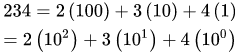Step:2 To round of the number nearest to tenth, analyze the coefficient of.

Step:3 If coefficient ofis greater than 5 then increase the coefficient ofand eliminate the coefficientsotherwise only eliminate the coefficients, to round of the number nearest to tenth.

Step:4 Here the coefficient ofis 4, which is less than 5, therefore eliminate the coefficients, to round of the number nearest to tenth as follows:Thus, the number 234 round to the nearest tenth is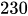.

The number 234 round to the nearest tenth is.

Problem 2:

Write the number 5400 to nearest tenth.

Solution:

Step:1 The given number is 5400.

expand the number in power of 10 as shown below: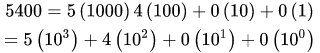Step:2 To round of the number nearest to tenth, analyze the coefficient of.

Step:3 If coefficient ofis greater than 5 then increase the coefficient ofand eliminate the coefficientsotherwise only eliminate the coefficients, to round of the number nearest to tenth.

Step:4 Here the coefficient ofis 0, which is less than 5, therefore eliminate the coefficients, to round of the number nearest to tenth as follows:Thus, the number 5400 round to the nearest tenth is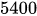.

The number 5400 round to the nearest tenth is.

### Need homework help now?

tutors online

Related Questions
##### (t) in °C of the pot of soup at time t (in The initial temperature of a pot of soup is 80°C. The temperature minutes) satisfies the differential equation dT di T-20 10 (a) Solve the differential equation, and find an explicit expression for the function (). By separating variables, integrating and manipulating arbitrary constants, . We are also told that so that and so . ...
Notes
Me

OR

Don't have an account?

Join OneClass

Access over 10 million pages of study
documents for 1.3 million courses.

Join to view

OR

By registering, I agree to the Terms and Privacy Policies
Just a few more details

So we can recommend you notes for your school.